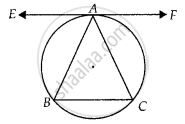# The tangent to the circumcircle of an isosceles triangle ABC at A, in which AB = AC, is parallel to BC. - Mathematics

MCQ
True or False

The tangent to the circumcircle of an isosceles triangle ABC at A, in which AB = AC, is parallel to BC.

• True

• False

#### Solution

This statement is True.

Explanation:

Let EAF be tangent to the circumcircle of ∆ABC.To prove: EAF ॥ BC

We have, ∠EAB = ∠ACB ......(i) [Angle between tangent and chord is equal to angle made by chord in the alternate segment]

Here, AB = AC

⇒ ∠ABC = ∠ACB  ......(ii)

From equation (i) and (ii), we get

∠EAB = ∠ABC

∵ Alternate angles are equal.

⇒ EAF ॥ BC

Concept: Concept of Circle - Centre, Radius, Diameter, Arc, Sector, Chord, Segment, Semicircle, Circumference, Interior and Exterior, Concentric Circles
Is there an error in this question or solution?

#### APPEARS IN

NCERT Mathematics Exemplar Class 10
Chapter 9 Circles
Exercise 9.2 | Q 7 | Page 105
Share